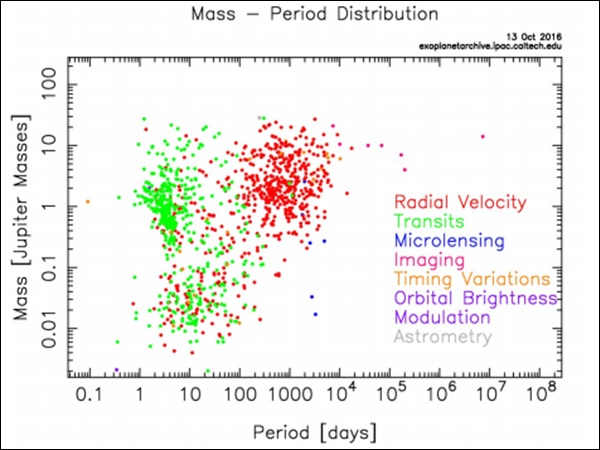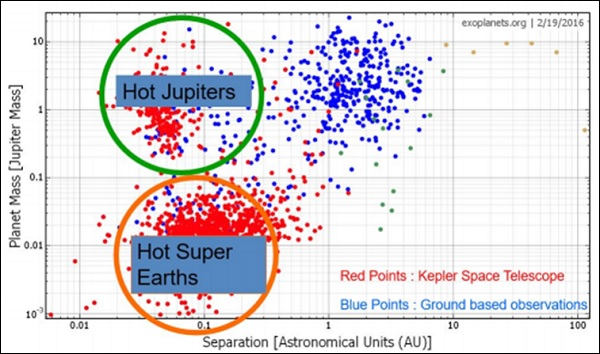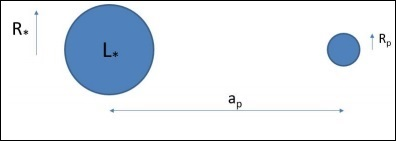# Cosmology - Exoplanet Properties

First direct image of an extrasolar planet, in 2004, was of a planet of mass 3-10 Mjupiter orbiting around a brown dwarf (2M1207) with a mass of 25 Mjupiter. Techniques like Radial velocity, Transit, Gravitational microlensing, Imaging, Astrometry, etc., has been used for detection of exoplanets. The number of detections have been increasing every year.

Until around 2010, the Radial velocity method was used extensively, but now most of the detections are done by the Transit method. There was a spike in the number of detections in 2014, which was when Kepler Space Telescope (KST) started giving the results.

A mass-period distribution shows that the Radial velocity method is more biased towards detection of massive planets with a larger period, whereas using the Transit method, planets with lower period are only detected as shown in the following image (Courtesy: NASA Exoplanet Archive).There is a colossal increase in the number of detection of smaller mass planets since the advent of KST. This is evident from the figure given below. The planets detected by KST are divided into two groups: hot massive planets called “Hot Jupiters” and lower mass planets called “Hot Super Earths” (as they are more massive than Earth).When we plot the number of extrasolar planets detected versus distance to them, we find that most of these planets are within 2kpc, which is well within our galaxy. Maybe the planets are not so uncommon in the universe, since our detection is limited to only certain type of planets in a very small part of the universe.

Planets are formed from circumstellar disc or proto planetary disc. If planets are formed as a by-product during star formation, maybe the number planets in the universe exceeds the number of stars in universe!!

## Habitable Zones

A Habitable Zone can be defined as the zone around the star where water can exist in its liquid form. Consider a planet at distance $a_p$ from the star as shown in the following figure. A simple method for calculating the temperature of the planet is described as follows.$$\left ( \frac{L_\ast}{4\pi a^2_p} \right )\pi R^2_p(1 - A) = 4\pi R^2_p \sigma T^4_p$$

and

$$\frac{L_\ast}{4\pi R^2_\ast} = \sigma T^4_\ast$$

$$\therefore T_p = (1 - A)T_\ast \sqrt{\frac{R_\ast}{2a_p}}$$

In our case substituting

• Lsun = 3.83 x 1026

• ap = 1.5 ∗ 1011 and

• A = 0.3

Will give $T_{Earth} = 255K$. The actual calculation is very involved which includes cloud physics. The Habitable zone in our solar system lies between 0.9 AU and 1.7 AU.

The Sun’s luminosity was found to be increasing with time because of the decreasing gas pressure. It was 30% less bright when it started burning hydrogen. This would result is shift of habitable zone away from the Sun. Since Earth is near the inner edge of the Habitable zone, maybe one day it will move out of the zone!

### Continuously Habitable Zone

In short it is termed as CHZ can be defined as the region in which liquid water can exist over the entire Main Sequence lifetime of a star. The KST has detected many extrasolar planets which do lie in the habitable zone.

A bio-signature is any substance − such as an element, isotope, molecule, or phenomenon that provides scientific evidence of past or present life. An example is detection of both O2 and CO2 on a planet, which is usually not possible through geological processes alone. This detection is done by analyzing the absorption spectra.

### Points to Remember

• Techniques like Radial velocity, Transit, Gravitational microlensing, Imaging, Astrometry, etc., have been used for detection of exoplanets.

• Radial velocity method is more biased towards detection of massive planets with larger period.

• Hot massive planets are called “Hot Jupiter’s” and lower mass planets are called “Hot Super Earths”.

• The number of planets in the universe exceeds the number of stars in the universe.

• A Habitable zone can be defined as the zone around the star where water can exist in its liquid form.